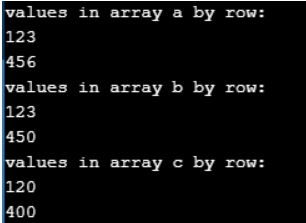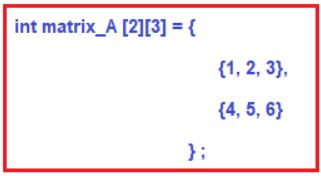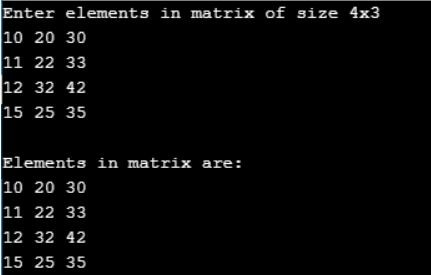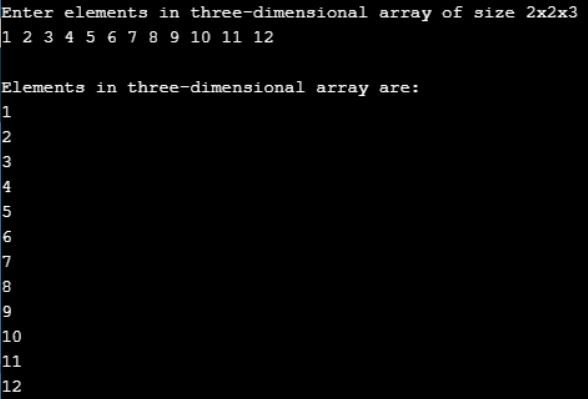# Multi-Dimensional Array in C

## Multi-Dimensional Array in C Language with Examples

In this article, I am going to discuss the Multi-Dimensional Array in C Language with Examples. Please read our previous articles, where we discussed One Dimensional Array in C Language with Examples.

##### Multi-Dimensional Array in C Language:

An array of arrays is called a multi-dimensional array. In simple words, an array created with more than one dimension (size) is called a multi-dimensional array. The multi-dimensional array can be of a two-dimensional array or three-dimensional array or four-dimensional array or more.

Syntax: type name[size1][size2]…[sizeN];
Example: int a;

###### Program to understand Multi-Dimensional Array in C Language:
```#include <stdio.h>
void arr(int x[]);  //function prototype
int main ()
{
int a = {{1,2,3}, {4,5,6}};   //initializing array
int b = {1, 2, 3, 4, 5};
int c = {{1, 2}, {4}};
printf("values in array a by row:\n");
arr(a);
printf("values in array b by row:\n");
arr(b);
printf("values in array c by row:\n");
arr(c);
return 0;
}             // end of main
void arr(int x[])
{
int i;      //row counter
int j;      //column counter
for (i = 0; i <= 1; ++i)
{
for (j = 0; j<= 2; ++j)
{
printf("%d", x[i][j]);
}       //end of inner for
printf("\n");
}          //end of outer for
}```
###### Output:The most popular and commonly used multi-dimensional array is a two-dimensional array. The 2-D arrays are used to store data in the form of a table. We also use 2-D arrays to create mathematical matrices.

##### Two-Dimensional Array in C Language:

The Two-dimensional array is nothing but a table with rows and columns. A two-dimensional array can be expressed as a contiguous and tabular block in memory where the data can be stored in a table structure.

Declaration of Two-Dimensional Array in C Language
Syntax: datatype arrayName [ rowSize ] [ columnSize ];

Initialization of Two-Dimensional Array in C Language
Syntax: datatype arrayName [rows][colmns] = {{r1c1value, r1c2value, …},{r2c1, r2c2,…}…};

Example: int matrix_A  = { {1, 2, 3},{4, 5, 6} };

The above declaration of two-dimensional array reserves 6 contiguous memory locations of 2 bytes each in the form of 2 rows and 3 columns. And the first row is initialized with values 1, 2 & 3 and the second row is initialized with values 4, 5 & 6. We can also initialize as follows:##### Accessing Individual Elements of Two-Dimensional Array in C Language

To access elements of a two-dimensional array we use the array name with the row index value and column index value of the element that is to be accessed. Here the row and column index values must be in separate square braces. In the case of the two-dimensional array, the compiler assigns separate index values for rows and columns.

Syntax: arrayName [ rowIndex ] [ columnIndex ];
Example: a = 20;
Here, the element with row index 0 and column index 1 of the array a is assigned a value of 10.

##### Implementation of Two-Dimensional Array in C Language:

A two-dimensional array can be implemented in two ways:

1. Row major implementation
2. Column major implementation
```#include <stdio.h>
#define ROW_SIZE 4 // Define constant row size
#define COL_SIZE 3 // Define constant column size
int main()
{
int matrix[ROW_SIZE][COL_SIZE];
int row, col;
printf("Enter elements in matrix of size %dx%d \n", ROW_SIZE, COL_SIZE);
/* Outer loop to iterate through each row */
for(row=0; row<ROW_SIZE; row++)
{
/* Inner loop to iterate through columns of each row */
for(col=0; col<COL_SIZE; col++)
{
/* Input element in array */
scanf("%d", &matrix[row][col]);
}
}
/* Print all elements of array */
printf("\nElements in matrix are: \n");
for(row=0; row<ROW_SIZE; row++)
{
for(col=0; col<COL_SIZE; col++)
{
printf("%d ", matrix[row][col]);
}
printf("\n");
}
return 0;
}```
###### Output:##### Points to Remember while working with 2D Array in C:
1. In a 2d array, elements are arranged in rows and columns format.
2. When we are working with the 2D array we require to use 2 subscript operators which indicate the row and column sizes.
3. In a 2D array when we are referring to one subscript operator then it gives the rows address, 2nd subscript operator gives the element
4. The main memory of the 2D array is rows and the sub-memory is columns.
5. On the 2D array, the array name always gives the main memory that is the 1st-row base address, arr+1 will give the next row base address.
##### Program for 3-D Array in C Language:
```#include <stdio.h>
#define SIZE1 2
#define SIZE2 2
#define SIZE3 3
int main()
{
int arr[SIZE1][SIZE2][SIZE3];
int i, j, k;
/*Input elements in array*/
printf("Enter elements in three-dimensional array of size %dx%dx%d \n", SIZE1, SIZE2, SIZE3);
for(i = 0; i < SIZE1; i++)
{
for(j = 0; j < SIZE2; j++)
{
for (k = 0; k < SIZE3; k++)
{
scanf("%d", &arr[i][j][k]);
}
}
}
/*Print elements of array*/
printf("\nElements in three-dimensional array are: \n");
for(i = 0; i < SIZE1; i++)
{
for(j = 0; j < SIZE2; j++)
{
for (k = 0; k < SIZE3; k++)
{
printf("%d\n", arr[i][j][k]);
}
}
}
return 0;
}```
###### Output:##### Applications of Arrays in C

In C Programming language, arrays are used in a wide range of applications. A few of them are as follows…

• Arrays are used to store a list of values: In C Programming language, single-dimensional arrays are used to store a list of values of the same datatype. In other words, single-dimensional arrays are used to store a row of values. In a single-dimensional array, data is stored in linear form.
• Arrays are used to Perform Matrix Operations: We use two-dimensional arrays to create a matrix. We can perform various operations on matrices using two-dimensional arrays.
• Arrays are used to implement Search Algorithms: We use single-dimensional arrays to implement search algorithms like Linear Search, Binary Search
• Arrays are used to implement Sorting Algorithms: We use single-dimensional arrays to implement sorting algorithms like Insertion Sort, Bubble Sort, Selection Sort, Quick Sort, Merge Sort, etc.,
• Arrays are used to implement Data structures: We use single-dimensional arrays to implement data structures like Stack Using Arrays and Queue Using Arrays